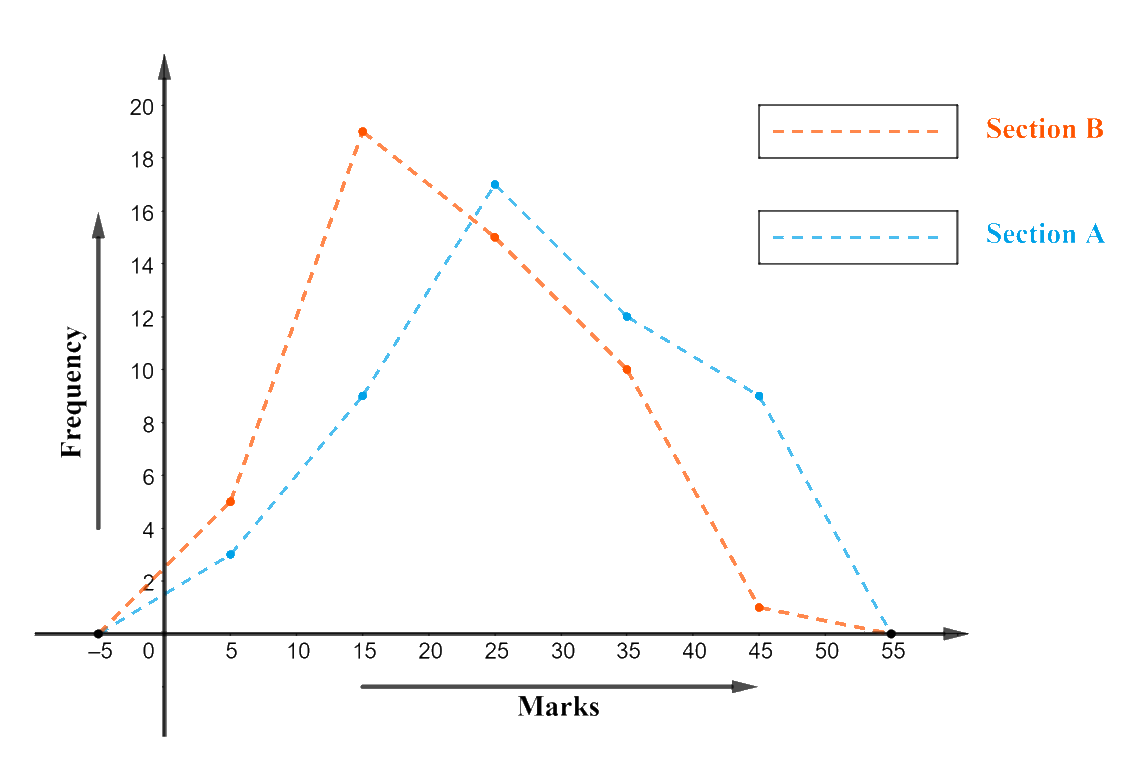# Ex.14.3 Q6 Statistics Solution - NCERT Maths Class 9

Go back to  'Ex.14.3'

## Question

The following table gives the distribution of students of two sections according to the marks obtained by them:

 Section A Section B Marks Frequency Marks Frequency $$0-10$$ $$3$$ $$0-10$$ $$5$$ $$10-20$$ $$9$$ $$10-20$$ $$19$$ $$20-30$$ $$17$$ $$20-30$$ $$15$$ $$30-40$$ $$12$$ $$30-40$$ $$10$$ $$40-50$$ $$9$$ $$40-50$$ $$1$$

Represent the marks of the students of both the sections on the same graph by two frequency polygons. From the two polygons compare the performance of the two sections.

Video Solution
Statistics
Ex exercise-14-3 | Question 6

## Text Solution

What is known?

The distribution of students of two sections according to the marks obtained by them.

What is Unknown?

(i) A frequency polygon to represent the marks of the students of both the sections.

(ii) Comparisons of performance of those two sections.

Reasoning:

Frequency polygons can also be drawn independently without drawing histograms. For this requires the midpoints of the class-intervals used in the data. The mid-points are called class-marks.

\text{Class}\,\text{Marks}=\frac{\left( \begin{align}& \text{Upper}\,\text{Limit+} \\ & \text{Lower}\,\text{Limit} \\ \end{align} \right)}{2}

Steps:

Now, the data table with inclusion of class marks:

 Section A Section B Marks Class Mark Frequency Marks Class Mark Frequency $$0-10$$ $$5$$ $$3$$ $$0-10$$ $$5$$ $$5$$ $$10-20$$ $$15$$ $$9$$ $$10-20$$ $$15$$ $$19$$ $$20-30$$ $$25$$ $$17$$ $$20-30$$ $$25$$ $$15$$ $$30-40$$ $$35$$ $$12$$ $$30-40$$ $$35$$ $$10$$ $$40-50$$ $$45$$ $$9$$ $$40-50$$ $$45$$ $$1$$

The frequency polygon for the above data can be constructed by:

• Taking class marks on $$x$$-axis.
• Taking ‘frequency’ on $$y$$-axis with an appropriate scale of $$1\,\rm unit = 2$$ students as the lowest frequency value observed is $$1$$ and the highest frequency value is $$19.$$It can be observed that the performance of students of Section ‘$$A$$’ is better than the students of Section '$$B$$' as section ‘$$A$$’ shows more students securing marks between ‘$$40 – 50$$’ class and ‘$$30 – 40$$’ class.

Learn from the best math teachers and top your exams

• Live one on one classroom and doubt clearing
• Practice worksheets in and after class for conceptual clarity
• Personalized curriculum to keep up with school